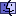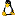Reading the Width and Height of a JPEG FileThis is a script which reads the width and height in a given JPEG file. I was planing to enable some weblog cgi scripts I made to handle photos and set the width and height parameters in HTML so that the images even if they are slightly bigger they will fit into a given rectangle and be scaled proportionally. True scaling of images usually requires extra software present on a server.

Here’s the script:

```on mouseUp
if it <> "" then
put GetDimensions(it)
-- puts <width>,<height>
end if
end mouseUp

function GetDimensions pFilePath
put url ("binfile:" & pFilePath) into rawFile
put length(rawFile) into tLen
put offset(numtochar("255") & numtochar("217"), rawFile) into eoj
if (eoj+2) >= tLen then put 0 into tCount
else put eoj into tCount
repeat while tCount<tLen
put offset(numtochar("255"), rawFile,tCount) into tOff
put chartonum(char tCount+tOff+1 of rawFile) into tChar
if tChar is among the items of "192,193,194,195" then
put char (tCount+tOff+5) to (tCount+tOff+8) of rawFile into rawSize
put baseconvert(chartonum(char 1 of rawSize),10,16) & baseconvert(chartonum(char 2 of rawSize),10,16) into hSize
put baseconvert(chartonum(char 3 of rawSize),10,16)& baseconvert(chartonum(char 4 of rawSize),10,16) into wSize
put "H=" & baseconvert(hSize,16,10) & "," & "W=" & baseconvert(wSize,16,10) into dimensions
return dimensions
end if